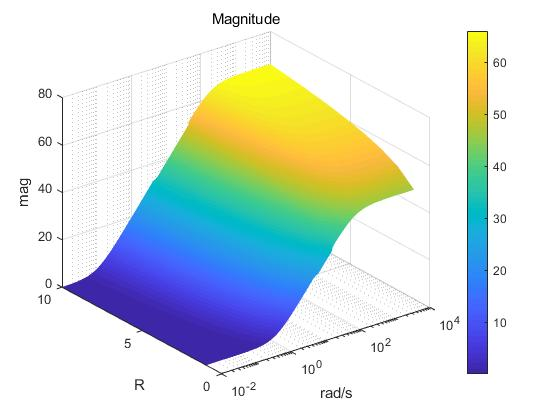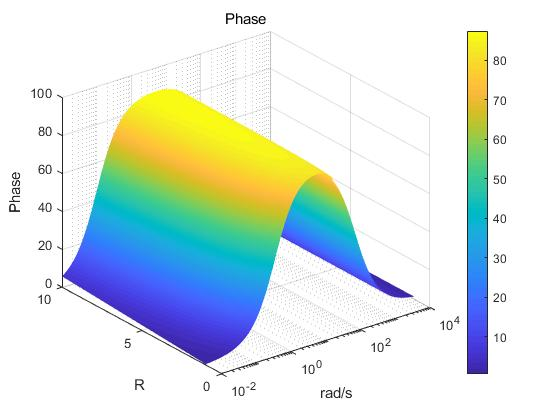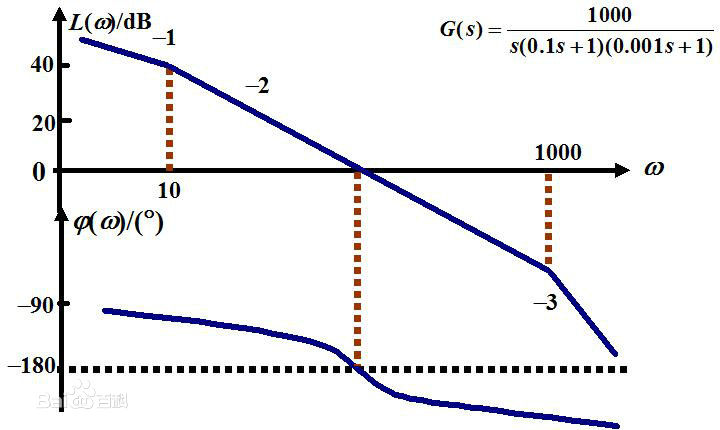• ## 伯德图（Bode图）分析系统性能

千次阅读 多人点赞 2020-02-19 19:19:31
根据Bode图，从系统频率的角度分析系统性能。 坐标系 伯德图由两张图组成，一个是幅频特性曲线，另一个是相频特性曲线。 伯德图横坐标为对数刻度，纵坐标幅值或相角采用线性分度。 幅频特性曲线，其中横坐标上为ω\...
伯德图分析系统性能1. 基本概念2. 伯德图绘制3. 系统分析3.1 开环频率与闭环系统性能的关系3.2 相位裕量 & 幅值裕量3.3 三段频分析系统性能3.4 其他指标对系统的影响
1. 基本概念

定义
伯德图是系统频率响应的一种图示方法。也称为开环对数频率特性曲线。
作用
根据Bode图，从系统频率的角度分析系统性能。
坐标系
伯德图由两张图组成，一个是幅频特性曲线，另一个是相频特性曲线。
伯德图横坐标为对数刻度，纵坐标幅值或相角采用线性分度。
幅频特性曲线，其中横坐标上为$\omega$，单位为$rad/s$，刻度为对数刻度，按照$\lg{\omega}$刻度；纵坐标为$20 \lg{|G(j\omega)|}$，单位为分贝（dB），按照线性刻度。
相位特性曲线，其中横坐标上为$\omega$，单位为$rad/s$，刻度为对数刻度，按照$\lg{\omega}$刻度；纵坐标为$\varphi(\omega)$，单位为度（°）按照线性刻度。

2. 伯德图绘制
绘制伯德图的一般步骤为：首先将开环频率特性改写为基本环节的乘积，画出各基本环节的伯德图，然后把各基本环节伯德图的对数幅值相加，相角相加，就得到系统的伯德图。
其中基本环节有：

比例环节；
惯性环节；
一阶微分环节；
积分环节；
微分环节；
振荡环节；
二阶微分环节；
延迟环节。

伯德图幅频特性曲线绘制的具体步骤：

确定系统开环增益$K$，并计算$20\lg{K}$；
确定各个具有转折频率环节的转折频率，标在坐标轴上；
在坐标轴上找出横坐标 $\omega =1$，纵坐标为 $20\lg{K}$的A点；
过A点做一直线，使其斜率等于-20vdB/十倍频程。当v=0, v=1, v=2时，斜率分别是(0，-20，-40)/十倍频程；
从低频段第一个转折频率开始做斜直线，该直线的斜率等于过A点直线的斜率加这个环节的斜率（惯性环节加-20，振荡环节加-40，一阶微分环节加+20的斜率），这样过每一个转折频率都要进行斜率的加减；
频段最后的斜线的斜率应等于-20(n-m) dB/十倍频程；
若系统中有振荡环节，当 $\xi<0.4$ 时，需对$L(\omega)$进行修正。

伯德图相频曲线绘制的具体步骤：

绘制各个环节的相频曲线；
各个环节的相频曲线相加；
当 $\omega\to0$   时，$\varphi(\omega)\to-v\cdot90\degree$
当 $\omega\to\infty$ 时，$\varphi(\omega)\to-(n-m)\cdot90\degree$

3. 系统分析
3.1 开环频率与闭环系统性能的关系
对于反馈系统来说，如下：开、闭环传递函数之间的关系为
$G_{B}(s)=\frac{G}{1+G_{K}}=\frac{G}{1+GH}$
其中：$G_{B}$是闭环传递函数，$G_{K}$是开环传递函数。
因此，在系统的结构、参数一定时，$G_{K}$也是一定的，那么闭环系统的动态响应和稳态性能就为一定。因此，就能够通过分析开环频率特性来了解系统的闭环响应性能。
奈氏判据就是一种利用开环特性来研究闭环性能的理论。这是一种利用复变函数中的辐角原理，建立判别系统稳定与否的一种方式。
由相位裕量、幅值裕量、截止频率、三段式等指标和方式判别系统稳定性的结论均是由奈氏判据推导而来。由于推导过程比较复杂，这里不做详述。做想做进一步了解，可参考《自动控制原理》（刘丁主编）这本书第五章内容。
3.2 相位裕量 & 幅值裕量

原始定义
相位裕量：开环幅频曲线幅值为1对用的相角值加上180°，称为$\gamma$ 。
幅值裕量：开环幅相曲线与负实值交点处的模值$|G(j\omega_{g})|$的倒数，称为$h$。
截止频率：一般指幅频截止频率，Bode图幅频曲线与横轴交点的频率，称为 $\omega_{c}$。
相频截止频率：Bode图相频曲线与-180°线交点的频率，称为 $\omega_{g}$。

物理意义
相位裕量：如果系统对频率信号 $\omega_{c}$ 相位再滞后 值，系统就处于临界稳定状态。
幅值裕量：如果系统的开环放大系数增大到原来的$h$倍，则闭环系统就进入临界稳定状态。而在应用中， 幅值裕量常常用分贝值$L_{h}$表示。
$L_{h} = 0 - 20\lg{|G(j\omega_{g})|}$

由伯德图计算方法
相位裕量：伯德图上截止频率对应的相位曲线上的角度与-180的差值。
幅值裕量：伯德图幅频曲线上横轴与相位截止频率对应幅频曲线值的差值。

系统分析
相位裕量：$\gamma>0$则系统稳定，否则系统不稳定。$\gamma$ 值越大，其系统的稳定程度越高，工程上一般要求 $\gamma≥40\degree(40\degree-60\degree)$ 。
幅值裕量：$L_{h}>0$则系统稳定，否则系统不稳定。$L_{h}$值越大，其闭环系统稳定程序越高。一般要求$L_{h} \geqslant6 dB(6 dB-10 dB)$ 。

3.3 三段频分析系统性能
低频段是指伯德图在第一个转折频率之前的区间，该段区间由开环增益和积分环节决定；中频段是指Bode图在截止频率 $\omega_{c}$ 附近的区间；高频段是指频率 $\omega > 10\omega_{c}$的区间。

低频段与系统稳定精度的关系
该低频段的斜率愈小，位置愈高，对应于系统积分环节的数目愈多，开环增益K值愈大。故其闭环系统在满足稳定的条件下，其稳态误差愈小，系统的稳态精度愈高。
中频段与系统动态性能的关系
该中频段斜率小于-60，则很难使闭环系统稳定；若等于-40，所占频率区间不宜过宽，则闭环系统可能稳定，即使稳定，其相稳定裕度也较小，系统的平稳性较差；如果中频段斜率为-20，且占据较宽的频段区间，一般说来，不仅可以保证系统稳定，而且可以使相稳定裕度增大，取得较好的平稳性。同时以提高截止频率来保证系统要求的快速性。
高频段与系统抗干扰能力
系统开环对数幅频在高频段的幅值，直接反映了系统对输入高频干扰信号的抑制能力。高频特性的分贝值愈低，系统抗干扰能力愈强。

3.4 其他指标对系统的影响

带宽
系统跟踪正弦输入信号，输出信号的幅值下降到和输入幅值的某一个比例时的频率。
在系统中，高频信号体现的是信号的变换快慢，一个信号中如果高频信号幅值高，则这个信号变换速度也快。所以如果一个系统的带宽低，虽然输入频率还是在带宽范围内，那么这个系统在响应变换快速信号时（比如阶跃），他的输出就不能响应的变化速度快，会有一个平滑的过程，并且过程长幅值低，及动态指标差（响应时间等）。反之，如果系统的带宽高，则可以动态性能好，但此时会影响幅值裕量和相位裕量，影响系统的稳定性能。所以，一个系统的带宽需要高，但不能太高。
截止频率
截止频率根据定义，是指增益为1的时候对应频率，那么其分析规律和带宽是一致的。


展开全文算法 线性代数 经验分享
• 做了一个简单的小例子，有什么疑惑可以留言。 clc clear close all %% 初始化 R_Sta=1; %R的起始，结束范围以及间隔 R_end=10; Nap_R=1; % 数组初始化 num=[R_Sta 1];...[mag,phase,wout]=bode(num,den,w);...
做了一个简单的小例子，有什么疑惑可以留言。

clc
clear
close all
%% 初始化
R_Sta=1;    %R的起始，结束范围以及间隔
R_end=10;
Nap_R=1;
% 数组初始化
num=[R_Sta 1];
den=[5e-4 1];
[mag,phase,wout]=bode(num,den,w);   % 先要运行一次bode函数以确定输出量的长度

R=R_Sta:Nap_R:R_end;
Num_R=length(R);
Num_w=length(wout);
Z_mag = ones(Num_R,Num_w);
Z_phase = ones(Num_R,Num_w);
[X,Y]=meshgrid(wout,R);

R=R_Sta;
for i=1:Num_R
num=[R 1]
den=[5e-3 1]
[mag,phase,wout]=bode(num,den,w);
Z_mag(i,:)=20*log10(mag);
Z_phase(i,:)=phase;
R=R+Nap_R;
end

% figure(1)
% bode(num,den);
figure(2)
C_mag=Z_mag;
surf(X,Y,Z_mag,C_mag)
set(gca,'XScale','log')
colorbar
title('Magnitude')
figure(3)
C_phase=Z_phase;
surf(X,Y,Z_phase,C_phase)
set(gca,'XScale','log')
colorbar
title('Phase')


输出图形如下：展开全文matlab
• ## bode图

千次阅读 2019-11-02 21:06:33
bode图横轴是频率，按照对数来刻度 1.横轴上标的10，100，对应的长度实际上是lg10，lg100对应得长度 2.等距等比： 3.频率等于0得地方在负无穷远处 4.很详细得展示低频段的信息，同时又尽可能的显示高频段 纵轴是...bode图横轴是频率，按照对数来刻度
1.横轴上标的10，100，对应的长度实际上是lg10，lg100对应得长度
2.等距等比：
3.频率等于0得地方在负无穷远处
4.很详细得展示低频段的信息，同时又尽可能的显示高频段
5.w是角频率（弧度/秒）

纵轴是幅值和相位，按照线性刻度

L(w) = 20lg(G(jw))

分贝(db)单位的由来
贝尔是声音领域的单位，$lg\frac{P_c}{P_r}$(贝尔),
$P_r$是人耳能听到的最微小的声音（每平方米10~20w）
一般我们乘以10叫做分贝，$10lg\frac{P_c}{P_r}$(分贝),
为啥要对比值取对数呢？
声音功率线性增长后，人耳听到的声音不是线性增长，而是对数增长的。

电学中db单位的由来
设一个系统输入功率是 $P_r=\frac{U_r^2}{R}$,输出功率是 $P_c=\frac{U_c^2}{R}$。
$10lg\frac{P_c}{P_r}=10lg\frac{U_c^2}{U_r^2}=20lg\frac{U_c}{U_r}$


展开全文Matlab中用Simulink快速画Bode图及 .m 文件画Bode图Simulink画Bode图.m 文件画Bode图
一万年没用matlab画过Bode图了，但是以后应该是要经常用到的，所以掏出自控原理，再学一遍Bode图的画法。通过代码来画实在是脑阔疼（不过习惯后应该还是会很得心应手的吧），经过师兄指点，发现了一个简单又快捷的画法，特此记录。然后右键传递函数（上图中为 Transfer Fcn2），选择 Linear Analysis，选择 Linearize Block，如下图所示：然后出现下图，点击右上方的 bode 选项即大功告成了。对于其他传递函数的 bode 图，可以同样的方式操作，最后选择 Bode Plot 1 则会在 Bode Plot 1 中继续画出新的 Bode 图，如下如示，这样就可以轻松比对各个 Bode 图了。若继续选择 Bode ，则会另开一个 Bode Plot 2 画出新的 Bode 图。对于Bode图中的数据，显然就在左边"Linear Analysis Workspace"中的"linsys1"和"linsys2"中，想提取出来再用其他画图工具自行绘制的可以用鼠标选中比如"linsys1"，将其拖到左上方的"MATLAB Workspace"中，这样我们就能在Matlab的workspace中找到该变量了，再通过如下语句即可获得幅频、相频数据：
>> [mag,phase,freq] = bode(linsys1)

.m 文件画Bode图
这个没什么好说的，无非就是几个函数的使用，所以直接上代码：
Hz = logspace(-1, 5, 10000);
w = 2 * pi * Hz;

num = [2 * pi * 25];
% den = [1 0];
% num = [2 * pi * 25];
den = [1 2 * pi * 25];

% bode(num, den, w);

[mag, pha, w1] = bode(num, den, w);
% log10w1 = log10(w1);
mag_plot = 20 * log10(mag);

subplot(2, 1, 1);
semilogx(Hz, mag_plot);
% plot(log10w1, mag_plot)

subplot(2, 1, 2);
semilogx(Hz, pha);
% plot(log10w1, pha);



展开全文• 在工程实践中,广泛应用经典控制理论的频域分析对自动控制系统进行分析和设计.Bode图是频域分析的重要环节,系统的闭环频域指标能够根据Bode图计算得出,从而分析自动控制系统闭环稳定性、快速性、准确性.传统手工绘制...
• 上标出任意一点即能找到其他闭环极点并算出K 根据输入的Kg找出闭环极点 num=[1 1];%华工课本P186 den=[1 3 12 -16 0]; axis equal; rlocus(num,den); title('根轨迹绘制') [k,p]=rlocfind(num,den)%人为选定点，...matlab
• 本代码是matlab 里面的.m文件，可以用来分析列好的传递函数的bode图，进而判断其稳定区域和剪切频率等。可以用来判断系统的稳定裕度。
• 一、拉氏变换 matlab自带工具：拉氏变换（laplace） 例laplace（f）返回f的拉普拉斯变换。 默认情况下，自变量是t，变换变量是s。 例laplace（f，transVar）使用转换变量transVar而不是s（自定义变换变量）。...matlab
• 用matlab画bode图画bode图的小练习可以直接运行的bode图参考网页 画bode图的小练习 用GM表示传递函数 GM=Wm/Tm 把wa，wb 代入GM 可以直接运行的bode图 第一个是margin方法，第二个用bode的方法。tf的使用...matlab
• 首先画出系统传函的bode图， 幅频曲线A(ω)与bode图的横轴ω的交点为(ωc, 0)，相频曲线φ(ω)与ω=-180°这条横线的交点为（ωg, -180°） 相位裕度的定义为：φ(ωc)-(-180°) 幅值裕度的定义为：0-A(ωg) ...
• ## matlab画BODE图GUI设计

千次阅读 2019-05-06 15:09:14
之前本科毕业设计是系统辨识与控制，用matlab设计了一个绘制系统BODE图的gui，在这里以作记录。 处理数据是系统的输入和输出时域数据，输入信号有正弦扫频信号和PRBS信号，使用FFT计算频域特性，效果图如下。 ...matlab gui fft
• ## matlab绘制bode图2

千次阅读 2020-07-13 16:30:38
Bode图由对数幅频特性和对数相频特性两张图构成，轴采用对数分度，而幅值为对数增益即分贝，相位为线性分度。下边利用MATLAB直接画。 例如： >> num =[5*0.1,1]; >> f1=[1,0];f2=[0.5,1]; >>...
• 然后在inport和outport之间使用传递函数（想要查看bode图的传函）连接 按如下路径点击Analysis——Control Design——Linear Analysis 在弹出的新界面中在Analysis I/Os中选择Root level inports and outports 在...
• 定性的分析系统的性能的时候，通常将bode图分为高、中、低三个频段，频段的分割也是相对的，但是不影响具体分析。 1、中频段一般是比较关键的，涉及到系统能否稳定等问题。比如如果中频段是-20dB衰减，那么我们希望...
• 2019独角兽企业重金招聘Python工程师标准>>> ...
• 分析k分别为4,1,0.5时bode图和阶跃响应的不同。 >> w=2*pi*50; >> num1=0.5*w*[1 0]; >> den1=[1 0.5*w w^2]; >> num2=1*w*[1 0]; >> den2=[1 1*w w^2]; >> num3=4*w*[1 ...matlabmatlab simulink
• 1.c2d()函数的用法 c2d()函数的作用是将s域的表达式转化成z域的表达式，s=0对应z=1。 c2d()函数转化的方法有多种： ①zoh, 零阶保持器法，又称阶跃响应不变法； ②foh ，一阶保持器法 ...③tustin ，双线性变换法 ...Matlab
• 我们经常会遇到使用Matlab画伯德图的情况，可能我们我们都知道bode这个函数是用来画bode图的，这个函数是Matlab内部提供的一个函数，我们可以很方便的用它来画伯德图，但是对于初学者来说，可能用起来就没有那么方便...
• 代码如下: g=tf(,[1,1]) P=bodeoptions; P.FreqUnits='Hz'; bode(g,P) 效果如下:
• 记录目的：学会如何正确的分析Bode图，并应用到在实际驱动调试中。 反思： 一、Bode图的认识 直观上看，Bode图中有2条曲线。 1、幅频（幅值vs频率） 特性曲线 上半部分绘制的曲线是：因变量Amplitude(幅值，或者...pid 控制器
• 现实中，常用的PI值都是正数，这是因为调整的正方向为正向，即误差增大，控制量增大的规律特性，但并不是所有的控制系统都是这种控制规律，有的是误差增大，控制量需要减小，这是PI的值就为负值了，或者说用一个恒定...